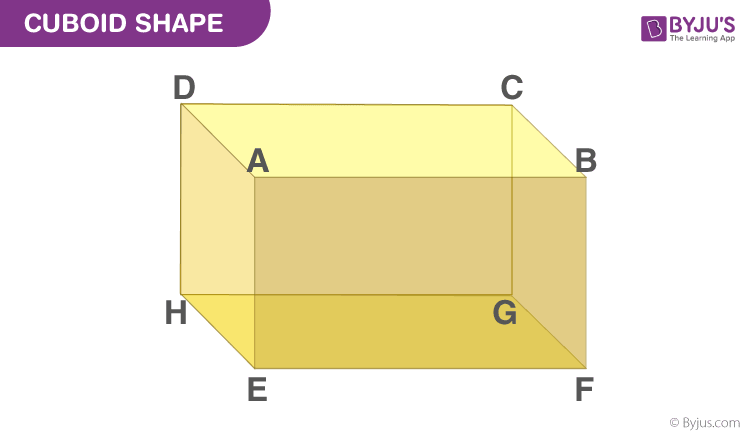# Visualise the area of a cuboid?

In geometry, a convex polyhedron that is bounded by six rectangular faces with eight vertices and twelve edges is called a cuboid. A cuboid has 6 faces, 8 vertices and 12 edges. All these can be shown using notation as given below.## Faces of cuboid

A Cuboid is made up of six rectangular faces. In the above-given figure, the six faces are:

• ABFE
• DAEH
• DCGH
• CBFG
• ABCD
• EFGH

The pair of opposite and parallel faces of the given cuboid are:

ABCD andEFGH (top and bottom faces respectively)

ABFE, DCGH, and DAEH, CBFG (opposite and parallel faces which are adjacent to top and bottom faces of the cuboid)

For each face, we can write the remaining faces as their adjacent faces such as the face ABCD is the adjacent face to ABFE, BCGF, CDHG, and ADHE.

## Edges of cuboid

The sides of all the rectangular faces are referred to as the edges of the cuboid. As we know, there are 12 edges for a cuboid. They are AB, AD, AE, HD, HE, HG, GF, GC, FE, FB, EF and CD respectively. Also, the opposite sides of a rectangle are equal. Therefore,

• AB = CD = GH = EF
• AE = DH = BF = CG
• EH = FG = AD = BC

## Vartices of cubiod

The point of intersection of the 3 edges of a cuboid is called the vertex of a cuboid and a cuboid has 8 vertices.

From the given cuboid figure, the 8 vertices are A, B, C, D, E, F, G and H.

## Cuboid surface area

Let l, b and h be the length, breadth and height of a cuboid respectively.

AB = CD = EF = GH = l

BC = AD = EH = FG = b

CG = BF = AE = DH = h

Lateral surface area of the cuboid = Area of face ADHE + Area of face BCGF + Area of face ABFE + Area of face DCGH

= (AD × DH) + (BC × CG) + (AB × BF) + (CD × BC)

= (b × h) + (b × h) + (l × h) + (l × h)

= 2(b × h) + 2(l × h)

Lateral surface area of the cuboid = 2h(l + b) sq.units

The total surface area (TSA) of a cuboid is the sum of areas of all its rectangular faces.

Area of face EFGH = Area of Face ABCD = (l × b)

Area of face BFGC = Area of face AEHD = (b × h)

Area of face DHGC = Area of face ABFE = (l × h)

Total surface area of a cuboid = Sum of the areas of all its 6 rectangular faces

= Area of (ABCD + EFGH + BFGC + AEHD + DHGC + ABFE)

= (l × b) + (l × b) + (b × h) + (b × h) + (l × h) + (l × h)

= 2lb + 2bh + 2hl

Total surface area of a cuboid = 2(lb + bh + hl) sq.units(0)(0)